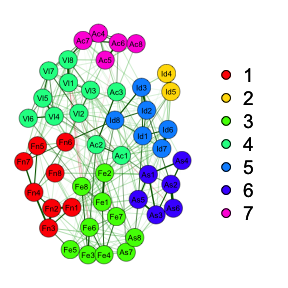# Introduction

This vignette shows you how to compute network scores using the state-of-the-art psychometric network algorithms in R. Building on the example proposed by Christensen (2018), this algorithm is now more efficient and precise given recent findings in the psychometric network literature. The vignette will walkthrough an example that compares latent network scores to latent variable scores computed by confirmatory factor analysis (CFA) and will explain the similarities and differences between the two.

To get started, a few packages need to be installed (if you don’t have them already) and loaded.

# Install 'NetworkToolbox' package
install.packages("NetworkToolbox")
# Install 'lavaan' package
install.packages("lavaan")
# Load packages
library(EGAnet)
library(NetworkToolbox)
library(lavaan)

# Estimate Dimensions

To estimate dimensions, we’ll use exploratory graph analysis (EGA; Golino, 2019; Golino & Epskamp, 2017; Golino et al., 2018). EGA first computes a network using either the graphical least absolute selection and shrinkage operator (GLASSO; Friedman, Hastie, & Tibshirani, 2008) with extended Bayesian information criterion (EBIC) from the R package qgraph (GeLASSO; Epskamp & Fried, 2018) or the triangulated maximally filtered graph (TMFG; Massara et al., 2016) from the NetworkToolbox package (Christensen, 2018). EGA then applies the walktrap community detection algorithm (Pons & Latapy, 2006) from the igraph package (Csardi & Nepusz, 2006). Below is the code to estimate EGA using the GeLASSO method with the NEO PI-3 openness to experience data (n = 802) in the NetworkToolbox package.

# Run EGA
ega <- EGA(neoOpen)As depicted above, EGA estimates there to be 7 dimensions of openness to experience. Using these dimensions, we can now estimate network scores; but first, I’ll go into details about how these are estimated.

Network loadings are roughly equivalent to factor loadings and differ only in the association measures used to compute them. For networks, the centrality measure node strength is used to compute the sum of the connections to a node. Previous simulation studies have reported that node strength is generally redundant with CFA factor loadings (Hallquist, Wright, & Molenaar, 2019) and item-scale correlations (Christensen, Golino, & Silvia, 2019). Importantly, Hallquist and colleagues (2019) found that a node’s strength represents a combination of dominant and cross-factor loadings. To mitigate this issue, I’ve developed a function called net.loads, which computes the node strength for each node in each dimension, parsing out the connections that represent dominant and cross-dimension loadings. Below is the code to compute standardized ($std; unstandardized, $unstd) network loadings.

# Standardized
net.loads <- net.loads(A = ega)\$std

To provide mathematical notation, first node strength must be defined:

$NS_i = \sum_j w_{ij},$ where $$w_{ij}$$ is the weight (e.g., partial correlation) between node $$i$$ and node $$j$$, and $$NS_i$$ is the sum of the weights between node $$i$$ and all other nodes. Notably, node strength must be defined for each community, leading to the following equation:

$NL_{iC} = \sum_{j \: \in \: C} NS_{ij},$

where $$NS_{ij}$$ is the node strength of node $$i$$ with the subset of nodes $$j$$ that belong to community $$C$$ (i.e., $$j \in C$$), and $$NL_{ik}$$ is the unstandardized network loading for node $$i$$ in community $$C$$. Finally, the standardized network loadings can be defined as:

$z_{NL_{iC}} = \frac{NL_{iC}}{\sqrt{\sum\limits_C NL_C}},$ where $$NL_C$$ is the sum of network loadings in community $$C$$, $$NL_{iC}$$ is the unstandardized network loading for node $$i$$ in community $$C$$, and $$z_{NL_{iC}}$$ is the standardized network loading of node $$i$$ in community $$C$$. It’s important to emphasize that network loadings are in the unit of association—that is, if the network consists of partial correlations, then the standardized network loadings are the partial correlation of each node with each dimension.

# Network Scores

These network loadings form the foundation for computing network scores. There are many, many ways for latent variable scores to be computed. In this formulation, these network scores correspond to the Maximum Likelihood estimation method of latent variable scores. Future development will expand network scores to include other estimation methods. Finally, it’s important to make clear that these scores are weighted composite scores, which means they are not truly a latent variable.

To compute network scores, the following code can be used:

# Network scores
net.scores <- net.scores(data = neoOpen, A = ega)

The net.scores function will return three objects: scores, commCor, and loads. scores contain the network scores for each dimension and an overall score. commCor contains the partial correlations between the dimensions in the network (and with the overall score). Finally, loads will return the standardized network loadings described above.

The network scores are computed following a partial least squares method. This starts by taking each community and identify items that do not have loadings on that community equal to zero, which for simplicity I’ll call $$z_{tC}$$:

$z_{tC} = z_{NL_{i \in C}} \neq 0,$ where $$t$$ represents an item in the community that does not have a loading equal to zero. After, $$z_{tC}$$ is divided by its standard deviation to obtain relative loadings for each item:

$rel_{tC} = \frac{z_{tC}}{\sqrt{\frac{\sum_{t=1}^n (z_{tC} - \bar{z_{.C}})^2}{n - 1}}},$ which can be further transformed into relative weights for each item:

$relWei_{tC} = \frac{rel_{tC}}{\sum_t rel_{tC}}$

Finally, these relative weights can be then be multiplied by the original data to obtain the community score:

$\hat{\theta_C} = \sum\limits_{t} X_{tC} \times relWei_{tC},$

where $$X$$ is the data, $$X_{tC}$$ are items, $$t$$, that do not have loadings on the factor, $$C$$, equal to zero, and $$\hat{\theta_C}$$ is the predicted network score for that community.